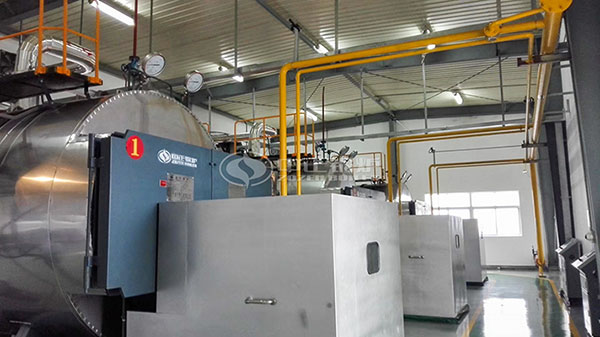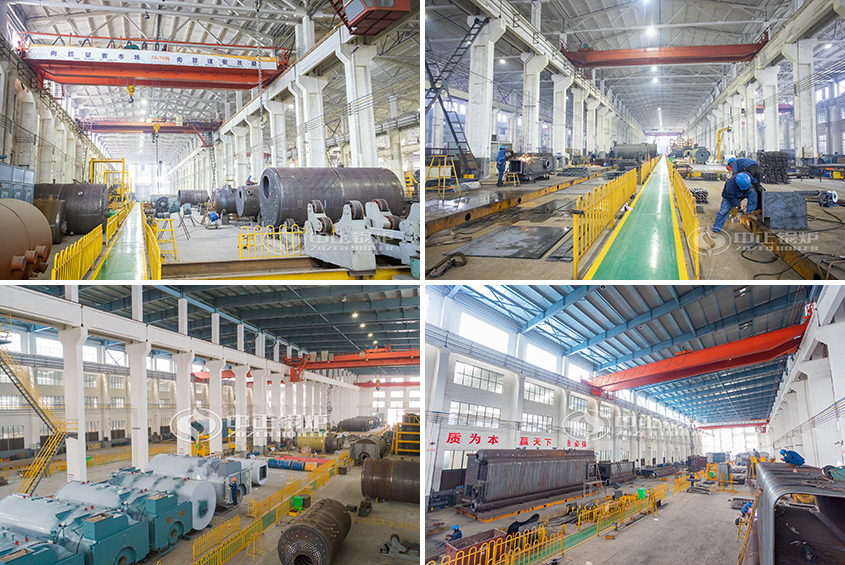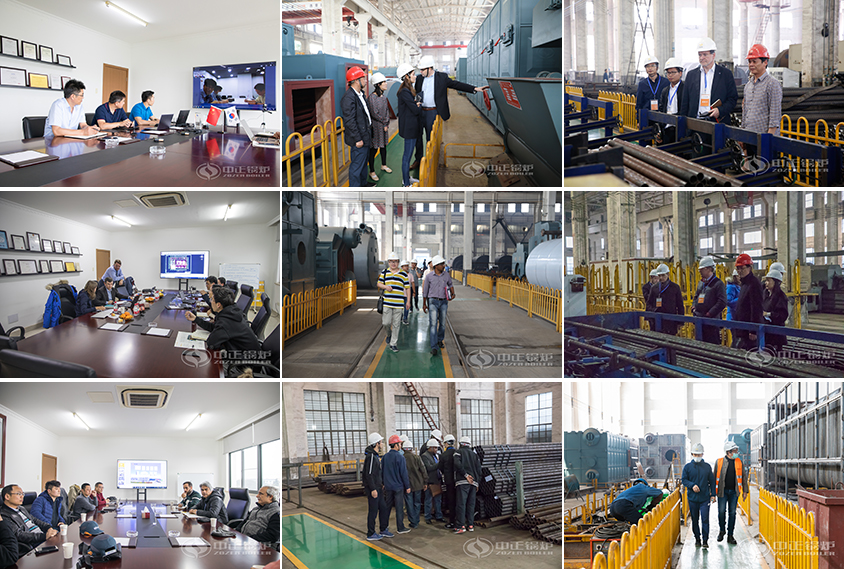# 1 KG Steam Cost for 1 Ton Steam Boiler

May 9, 2020

How much steam per cubic meter of natural gas produced by a 1 ton gas boiler is mainly affected by the calorific value and purity of the fuel and the thermal efficiency of the boiler? In addition, it has a close relationship with the operation level of the boiler maker.

1. Fuel calorific value. Because the quality of natural gas supply in different regions is different, the quality of gas boilers is different, the amount of mixed air is different, and the low calorific value of gas is also different. The calculation of the gas consumption of a gas boiler should specify the thermal efficiency value of the gas boiler. If the boiler thermal efficiency value is high, the gas consumption will be reduced, and vice versa.

2. The thermal efficiency of the boiler. When the fuel calorific value is unchanged, the boiler gas consumption is inversely proportional to the thermal efficiency. The higher the boiler thermal efficiency value, the less natural gas is used and the lower the cost. The thermal efficiency of the boiler itself is mainly related to the heating surface of the boiler, the convection heating area of the boiler, and the temperature of the exhaust gas. Reasonably control the exhaust gas temperature, reduce heat loss, and help users greatly reduce the daily operating cost of gas boilers.The cost of producing 1 kg of steam for a 1 ton gas steam boiler is:

1. Its rated evaporation capacity is 1 ton / hour, the consumption of natural gas fuel is 70 cubic meters, and the price of natural gas is calculated at 3.5 yuan / cubic (the price will vary slightly in different places), then the gas cost is: 70 * 3.5 = 245 yuan;

2. During normal use of gas-fired boilers, the electrical power is 0.65 times the full load power, then the electrical power of the boiler is (1.5kw + 2.2kw + 2kw) * 0.65 = 3.7kw, if the electricity fee is 1 yuan / degree Then the electricity cost per hour for the boiler is 3.7kw * 1 = 3.7 yuan;

3.  A 1 ton gas steam boiler can turn 1 ton of water into 1 ton of steam in an hour. In the middle, due to sewage and softened water treatment, etc., 1.1 tons of water is required to produce 1 ton of steam during normal use. Yuan / ton calculation, then the hourly water cost of gas boiler is: 1.1 * 3 = 3.3 yuan;

4. Because the gas boiler is a highly automated boiler, it does not require multiple people to take turns. A furnace worker can be used. If it is calculated based on a monthly salary of 2,000 yuan, it will work for 8 hours a day and the labor cost of the boiler is 2000 per hour /30/8=8.3 yuan;

5. The failure rate of Zhongzhen WNS gas boiler and SZS gas boiler is extremely low, and accordingly, the maintenance cost of the boiler is also low, so if it is a 1 ton gas steam boiler, the maintenance cost of 2000 a year will be enough. According to 365 days a year, 8 hours a day, the maintenance cost of the boiler per hour is 2000/365/8 = 0.68 yuan;

According to the above calculation, the total operating cost per hour for a 1 ton natural gas boiler is 245 + 3.7 + 3.3 + 8.3 + 0.68 == 252.6 yuan.

Also remind everyone that this is the boiler cost when running at full load, but usually the boiler will not run in this state. Therefore, the actual boiler operating cost budget is much lower than 252.6.

## Boiler Production Workshop## Customer Visiting